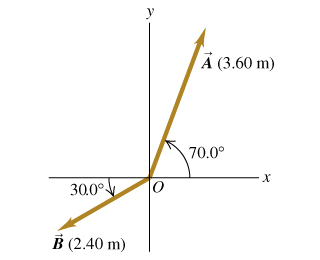# Problem: For the two vectors exttip{vec{A}}{A_vec} and exttip{vec{B}}{B_vec} in the figure , find the scalar product vec{A} cdot vec{B}.Find the magnitude of the vector product vec{A} imes vec{B}.What is the direction of the vector product vec{A} imes vec{B}?

⚠️Our tutors found the solution shown to be helpful for the problem you're searching for. We don't have the exact solution yet.

###### Problem Details

For the two vectors and in the figure, find the scalar product .

Find the magnitude of the vector product .

What is the direction of the vector product ?